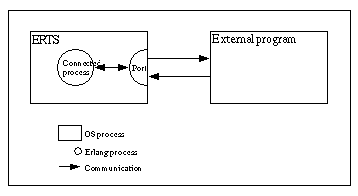User's Guide
PDF
Top

Interoperability Tutorial
User's Guide
Version 6.4

Expand All
Contract All

Chapters

• Introduction
• Overview
• Problem Example
• Ports
• Erl_Interface
• Port drivers
• C Nodes
• NIFs

# 4 Ports

This is an example of how to solve the example problem by using a port.Figure 4.1:   Port Communication.

### 4.1  Erlang Program

First of all communication between Erlang and C must be established by creating the port. The Erlang process which creates a port is said to be the connected process of the port. All communication to and from the port should go via the connected process. If the connected process terminates, so will the port (and the external program, if it is written correctly).

The port is created using the BIF open_port/2 with {spawn,ExtPrg} as the first argument. The string ExtPrg is the name of the external program, including any command line arguments. The second argument is a list of options, in this case only {packet,2}. This option says that a two byte length indicator will be used to simplify the communication between C and Erlang. Adding the length indicator will be done automatically by the Erlang port, but must be done explicitly in the external C program.

The process is also set to trap exits which makes it possible to detect if the external program fails.

```-module(complex1).
-export([start/1, init/1]).

start(ExtPrg) ->
spawn(?MODULE, init, [ExtPrg]).

init(ExtPrg) ->
register(complex, self()),
process_flag(trap_exit, true),
Port = open_port({spawn, ExtPrg}, [{packet, 2}]),
loop(Port).```

Now it is possible to implement complex1:foo/1 and complex1:bar/1. They both send a message to the complex process and receive the reply.

```foo(X) ->
call_port({foo, X}).
bar(Y) ->
call_port({bar, Y}).

call_port(Msg) ->
complex ! {call, self(), Msg},
{complex, Result} ->
Result
end.```

The complex process encodes the message into a sequence of bytes, sends it to the port, waits for a reply, decodes the reply and sends it back to the caller.

```loop(Port) ->
{call, Caller, Msg} ->
Port ! {self(), {command, encode(Msg)}},
{Port, {data, Data}} ->
Caller ! {complex, decode(Data)}
end,
loop(Port)
end.```

Assuming that both the arguments and the results from the C functions will be less than 256, a very simple encoding/decoding scheme is employed where foo is represented by the byte 1, bar is represented by 2, and the argument/result is represented by a single byte as well.

```encode({foo, X}) -> [1, X];
encode({bar, Y}) -> [2, Y].

decode([Int]) -> Int.```

The resulting Erlang program, including functionality for stopping the port and detecting port failures is shown below.

```
-module(complex1).
-export([start/1, stop/0, init/1]).
-export([foo/1, bar/1]).

start(ExtPrg) ->
spawn(?MODULE, init, [ExtPrg]).
stop() ->
complex ! stop.

foo(X) ->
call_port({foo, X}).
bar(Y) ->
call_port({bar, Y}).

call_port(Msg) ->
complex ! {call, self(), Msg},
{complex, Result} ->
Result
end.

init(ExtPrg) ->
register(complex, self()),
process_flag(trap_exit, true),
Port = open_port({spawn, ExtPrg}, [{packet, 2}]),
loop(Port).

loop(Port) ->
{call, Caller, Msg} ->
Port ! {self(), {command, encode(Msg)}},
{Port, {data, Data}} ->
Caller ! {complex, decode(Data)}
end,
loop(Port);
stop ->
Port ! {self(), close},
{Port, closed} ->
exit(normal)
end;
{'EXIT', Port, Reason} ->
exit(port_terminated)
end.

encode({foo, X}) -> [1, X];
encode({bar, Y}) -> [2, Y].

decode([Int]) -> Int.
```

### 4.2  C Program

On the C side, it is necessary to write functions for receiving and sending data with two byte length indicators from/to Erlang. By default, the C program should read from standard input (file descriptor 0) and write to standard output (file descriptor 1). Examples of such functions, read_cmd/1 and write_cmd/2, are shown below.

```
/* erl_comm.c */

typedef unsigned char byte;

{
int len;

return(-1);
len = (buf << 8) | buf;
}

write_cmd(byte *buf, int len)
{
byte li;

li = (len >> 8) & 0xff;
write_exact(&li, 1);

li = len & 0xff;
write_exact(&li, 1);

return write_exact(buf, len);
}

{
int i, got=0;

do {
if ((i = read(0, buf+got, len-got)) <= 0)
return(i);
got += i;
} while (got<len);

return(len);
}

write_exact(byte *buf, int len)
{
int i, wrote = 0;

do {
if ((i = write(1, buf+wrote, len-wrote)) <= 0)
return (i);
wrote += i;
} while (wrote<len);

return (len);
}
```

Note that stdin and stdout are for buffered input/output and should not be used for the communication with Erlang!

In the main function, the C program should listen for a message from Erlang and, according to the selected encoding/decoding scheme, use the first byte to determine which function to call and the second byte as argument to the function. The result of calling the function should then be sent back to Erlang.

```
/* port.c */

typedef unsigned char byte;

int main() {
int fn, arg, res;
byte buf;

fn = buf;
arg = buf;

if (fn == 1) {
res = foo(arg);
} else if (fn == 2) {
res = bar(arg);
}

buf = res;
write_cmd(buf, 1);
}
}

```

Note that the C program is in a while-loop checking for the return value of read_cmd/1. The reason for this is that the C program must detect when the port gets closed and terminate.

### 4.3  Running the Example

1. Compile the C code.

`unix> gcc -o extprg complex.c erl_comm.c port.c`

2. Start Erlang and compile the Erlang code.

```unix> erl
Erlang (BEAM) emulator version 4.9.1.2

Eshell V4.9.1.2 (abort with ^G)
1> c(complex1).
{ok,complex1}```

3. Run the example.

```2> complex1:start("extprg").
<0.34.0>
3> complex1:foo(3).
4
4> complex1:bar(5).
10
5> complex1:stop().
stop```